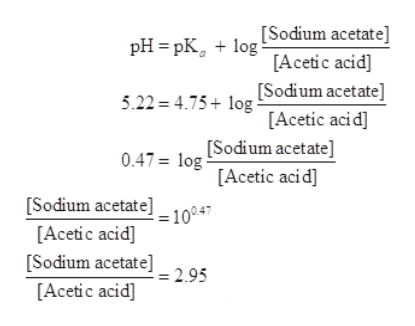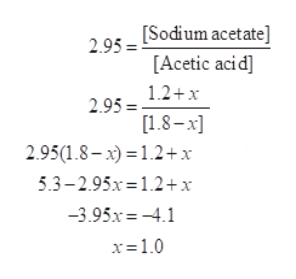# How much NaOH (in moles) must be added to 1 L of a buffer solution that a 1.8 M in acetic acid and 1.2 M in sodium acetate to result in buffer solution of pH 5.22. Assume volume to remain constant.

Question

How much NaOH (in moles) must be added to 1 L of a buffer solution that a 1.8 M in acetic acid and 1.2 M in sodium acetate to result in buffer solution of pH 5.22. Assume volume to remain constant.

check_circleExpert Solution
Step 1

A buffer solution is that solution which resists the change in its pH when small amount of acid or base is added to it. Acetate buffer is composed of weak acid and its salt with strong base in a specific concentration .

Step 2

As per question, the required pH of buffer is 5.22, concentration of acetic acid = 1.8 M, concentration of sodium acetate = 1.2 M

pKa of acetic acid = 1.8 x 10-5, pKa = -log (1.8 x 10-5) = 4.75

According to Henderson Hasselbalch equation for acetate buffer,help_outlineImage Transcriptionclose[Sodium acetate] pH 3 pК, + 1og [Acetic acid] 5.22 4.75log [Sodium acetate] [Acetic aci d] 0.47= log Sodium acetate] [Acetic acid] [Sodium acetate]=10047 [Acetic acid] [Sodium acetate]_2 95 [Acetic acid] fullscreen
Step 3

Let the moles of NaOH to be added = x

Required ratio of [sodium acetate]:[acetic acid] = 2.95

As the NaOH added will react with acetic acid to form sodium acetate, [sodium acetate] will increase while [acetic...help_outlineImage Transcriptionclose295 Sodium acetate] [Acetic acid] 1.2+x 2.95 = [1.8-x 2.95(1.8-x)12+ x 5.3-2.95x 1.2+x 3.95x-4.1 x-1.0 fullscreen

### Want to see the full answer?

See Solution

#### Want to see this answer and more?

Solutions are written by subject experts who are available 24/7. Questions are typically answered within 1 hour*

See Solution
*Response times may vary by subject and question
Tagged in

### Chemistry